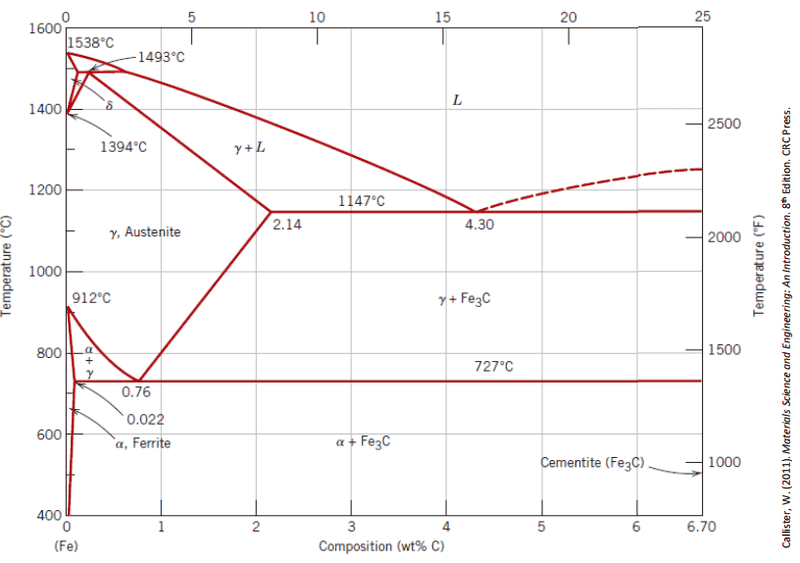# Phase Diagram of Steel with unknown C content

Knife

## Homework Statement

Given that the overall weight percentage of ferrite along the grain boundaries of an as-cast steel with unknown C amount is 35.23%:

a) Identify if it is hypoeutectoid or hypereutectoid.
b) Identify the amount of carbon alloyed in the steel.
c) Identify how much ferrite and cementite are in sample.
d) Identify the amount of eutectoid ferrite.

## Homework Equations## The Attempt at a Solution

I know that eutectoid is at 0.76 wt% C. If the wt% C is less it is hypoeutectoid, otherwise it is hypereutectoid. The problem is, the wt% C is unknown and I think I need to find that first to be able to answer a.

I'm given the weight percent of ferrite along the grain boundaries. I know there is a formula for computing the weight percentages using the lever rule, but you need to know the temperature in order to use the lever rule as far as our previous examples went, which is not given.

I really don't know how to start. The whole lecture is very muddy to me conceptually.

I don't think "as-cast" was mentioned in our lecture before, does this have any influence on the answer?

Knife
Update.

a) Since the ferrite is at the grain boundaries it can be assumed this is formed above 727 C. By inspecting the phase diagram: Hypoeutectoid begins at the austenite area, then goes to the ferrite and austenite area. Thus, the answer is hypoeutectoid.

b) I just know that the answer should be <0.76 wt% C. I still don't understand how to obtain this.

Update 2.

This is why I am stuck at b, if I use the lever rule:

$$W_{pro-\alpha} = \frac{0.76-A}{0.76-B}$$

A is the wt% C which I don't know, and B is at the solubility line which varies with T but I also don't know T.

Last edited: